# Fraction calculator

The calculator performs basic and advanced operations with fractions, expressions with fractions combined with integers, decimals, and mixed numbers. It also shows detailed step-by-step information about the fraction calculation procedure. Solve problems with two, three, or more fractions and numbers in one expression.

## Result:

### 3/7 + 1/4 = 19/28 ≅ 0.6785714

Spelled result in words is nineteen twenty-eighths.

### How do you solve fractions step by step?

1. Add: 3/7 + 1/4 = 3 · 4/7 · 4 + 1 · 7/4 · 7 = 12/28 + 7/28 = 12 + 7/28 = 19/28
For adding, subtracting, and comparing fractions, it is suitable to adjust both fractions to a common (equal, identical) denominator. The common denominator you can calculate as the least common multiple of both denominators - LCM(7, 4) = 28. In practice, it is enough to find the common denominator (not necessarily the lowest) by multiplying the denominators: 7 × 4 = 28. In the next intermediate step, the fraction result cannot be further simplified by canceling.
In words - three sevenths plus one quarter = nineteen twenty-eighths.

#### Rules for expressions with fractions:

Fractions - use the slash “/” between the numerator and denominator, i.e., for five-hundredths, enter 5/100. If you are using mixed numbers, be sure to leave a single space between the whole and fraction part.
The slash separates the numerator (number above a fraction line) and denominator (number below).

Mixed numerals (mixed fractions or mixed numbers) write as non-zero integer separated by one space and fraction i.e., 1 2/3 (having the same sign). An example of a negative mixed fraction: -5 1/2.
Because slash is both signs for fraction line and division, we recommended use colon (:) as the operator of division fractions i.e., 1/2 : 3.

Decimals (decimal numbers) enter with a decimal point . and they are automatically converted to fractions - i.e. 1.45.

The colon : and slash / is the symbol of division. Can be used to divide mixed numbers 1 2/3 : 4 3/8 or can be used for write complex fractions i.e. 1/2 : 1/3.
An asterisk * or × is the symbol for multiplication.
Plus + is addition, minus sign - is subtraction and ()[] is mathematical parentheses.
The exponentiation/power symbol is ^ - for example: (7/8-4/5)^2 = (7/8-4/5)2

#### Examples:

adding fractions: 2/4 + 3/4
subtracting fractions: 2/3 - 1/2
multiplying fractions: 7/8 * 3/9
dividing Fractions: 1/2 : 3/4
exponentiation of fraction: 3/5^3
fractional exponents: 16 ^ 1/2
adding fractions and mixed numbers: 8/5 + 6 2/7
dividing integer and fraction: 5 ÷ 1/2
complex fractions: 5/8 : 2 2/3
decimal to fraction: 0.625
Fraction to Decimal: 1/4
Fraction to Percent: 1/8 %
comparing fractions: 1/4 2/3
multiplying a fraction by a whole number: 6 * 3/4
square root of a fraction: sqrt(1/16)
reducing or simplifying the fraction (simplification) - dividing the numerator and denominator of a fraction by the same non-zero number - equivalent fraction: 4/22
expression with brackets: 1/3 * (1/2 - 3 3/8)
compound fraction: 3/4 of 5/7
fractions multiple: 2/3 of 3/5
divide to find the quotient: 3/5 ÷ 2/3

The calculator follows well-known rules for order of operations. The most common mnemonics for remembering this order of operations are:
PEMDAS - Parentheses, Exponents, Multiplication, Division, Addition, Subtraction.
BEDMAS - Brackets, Exponents, Division, Multiplication, Addition, Subtraction
BODMAS - Brackets, Of or Order, Division, Multiplication, Addition, Subtraction.
GEMDAS - Grouping Symbols - brackets (){}, Exponents, Multiplication, Division, Addition, Subtraction.
Be careful, always do multiplication and division before addition and subtraction. Some operators (+ and -) and (* and /) has the same priority and then must evaluate from left to right.

## Fractions in word problems:

• Fraction and a decimalWrite as a fraction and a decimal. One and two plus three and five hundredths
• Rolls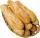Mom bought 13 rolls. Dad ate 3.5 rolls. How many rolls left when Peter yet put two at dinner?
• One third 2One third of all students in class live in a house. If here are 42 students in a class, how many of them live in house?
• Missing numberBlank +1/6 =3/2 find the missing number
• Akpan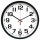Akpan spent 3/8 of his time in school during the week. What fraction of his time does he spend at home during the week?
• Land areaThe land area of Asia and Africa are in a 3: 2 ratio, the European and African are is 1:3. What are the proportions of Asia, Africa, and Europe?
• The book 4Mr. Kinion read 3 3/4 chapters in his book on Monday. He then read 2 4/6 more chapters on Tuesday. How many chapters has he read so far?
• Mixed numbersRewrite mixed numbers, so the fractions have the same denominator: 5 1/5 - 2 2/3
• Two thirdsTwo thirds of the unknown number is 8/7. What is 7/3 of this number?
• Fruits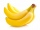For the price of one pineapple, I will buy two oranges. For the price of three oranges, I will buy four apples. I will buy 6 bananas for the price of three apples. What is the price of one banana if I pay 1 euro for one pineapple?
• Coin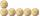How many 50-cent coins must be put in a row that next 50-cent coins after them (above) rolled on it axis?
• Two sisters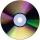Two sisters together have 54 CDs. 6/8 CDs has younger sister and is equal to 3/5 of CDs older sisters. How many CDs has each of the sisters?
• SavingsEva borrowed 1/3 of her savings to her brother, 1/2 of savings spent in the store and 7 euros left. How much did she save?